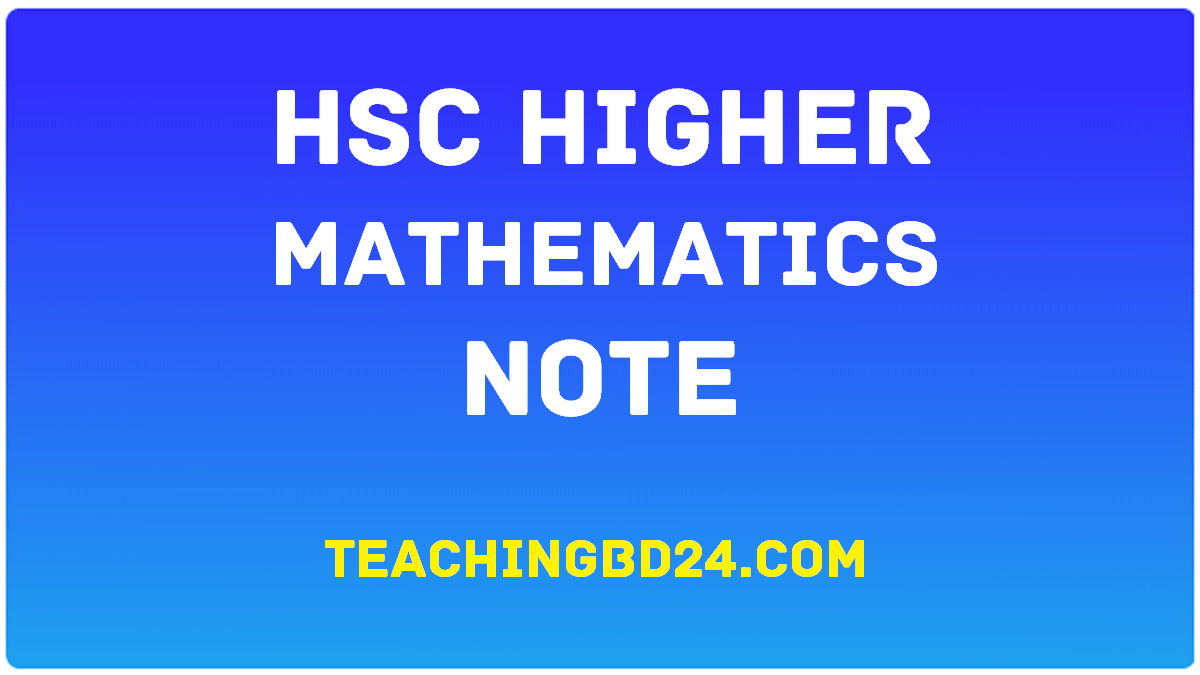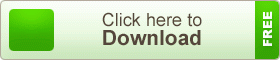# HSC Higher Mathematics 2nd Paper Note 1st Chapter Real numbers and Inequalities

HSC Higher Mathematics 2nd Paper Note 1st Chapter Real numbers and Inequalities. In mathematics, a real number is a value that represents a quantity along a line. The adjective real in this context was introduced in the 17th century by René Descartes, who distinguished between real and imaginary roots of polynomials. The real numbers include all the rational numbers, such as the integer −5 and the fraction 4/3, and all the irrational numbers, such as √2 (1.41421356…, the square root of 2, an irrational algebraic number). Included within the irrationals are the transcendental numbers, such as π (3.14159265…). Real numbers can be thought of as points on an infinitely long line called the number line or real line, where the points corresponding to integers are equally spaced. Any real number can be determined by a possibly infinite decimal representation, such as that of 8.632, where each consecutive digit is measured in units one-tenth the size of the previous one. The real line can be thought of as a part of the complex plane, and complex numbers include real numbers.

## HSC Higher Mathematics 2nd Paper Note 1st Chapter Real numbers and InequalitiesInequalities are governed by the following properties. All of these properties also hold if all of the non-strict inequalities (≤ and ≥) are replaced by their corresponding strict inequalities (< and >) and (in the case of applying a function) monotonic functions are limited to strictly monotonic functions. Any monotonically increasing function may be applied to both sides of an inequality (provided they are in the domain of that function) and it will still hold. Applying a monotonically decreasing function to both sides of inequality means the opposite inequality now holds. The rules for the additive inverse and the multiplicative inverse for positive numbers are both examples of applying a monotonically decreasing function. If the inequality is strict (a < b, a > b) and the function is strictly monotonic, then the inequality remains strict. If only one of these conditions is strict, then the resultant inequality is non-strict. The rules for additive and multiplicative inverses are both examples of applying a strictly monotonically decreasing function.

teachingbd24.com is such a website where you would get all kinds of necessary information regarding educational notes, suggestions and questions’ patterns of school, college, and madrasahs. Particularly you will get here special notes of physics that will be immensely useful to both students and teachers. The builder of the website is Mr. Md. Shah Jamal Who has been serving for 30 years as an Asst. Professor of BAF Shaheen College Dhaka. He expects that this website will meet up all the needs of Bengali version learners /students. He has requested both concerned students and teachers to spread this website home and abroad.